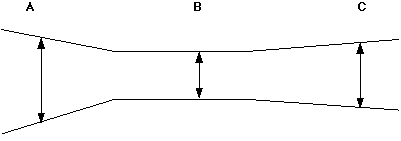+ Text Only Site
+ Non-Flash Version
+ Contact Glenn## Experiment on Fluids: Finding the Velocity of a Fluid in a Confined Container

SUBJECT: Aeronautics
TOPIC: Fluid Velocity
DESCRIPTION: A set of mathematics problems dealing with fluid velocity.
CONTRIBUTED BY: Carol Hodanbosi
EDITED BY: Jonathan G. Fairman - August 1996

#### Purpose:

To calculate the velocity of a confined fluid, given the cross-section area and velocity of another region.

#### Concept:

The drawing below is a cross-section of a circular cone attached to a circular cylinder.When a fluid (liquid or gas) is in a confined space, with no change in pressure or temperature, one can use the equation of continuity to find the velocity of the fluid if one knows the cross-section area and velocity in one of the regions. The formula for this is A1*V1 = A2*V2, where A is the cross-section area of one location and V is the velocity for that location.

#### Analysis:

Given three different locations in a confined container, A, B, and C, all having different radii, can you find the other two velocities of the fluid, if the velocity at A is given?

1. If the cross-section at A has a radius of 6 meters, can you find the area of the slice through the cone
(Area = pi * r 2)?

2. If the velocity of the fluid at location A is 10.0 m/s, and the radius at location B is 4.2 meters, can you find the velocity at location B?

3. If the velocity at location C is 8.6 m/s, can you find the radius at location C?

Extension:

4. If the radius of a fourth location, D, is one-half the radius of A , how would the velocity at location D compare to the velocity of the fluid at A? If D had one-third the radius of A, compare the velocity of the fluid at D to A. Explain your reasoning showing calculations.+ Inspector General Hotline + Equal Employment Opportunity Data Posted Pursuant to the No Fear Act + Budgets, Strategic Plans and Accountability Reports + Freedom of Information Act + The President's Management Agenda + NASA Privacy Statement, Disclaimer, and Accessibility CertificationEditor: Tom Benson NASA Official: Tom Benson Last Updated: May 13 2021 + Contact Glenn